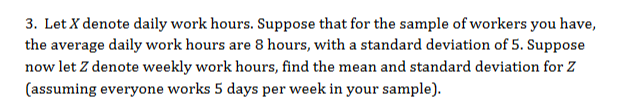# 3. Let X denote daily work hours. Suppose that for the sample of workers you have,the average daily work hours are 8 hours, with a standard deviation of 5. Supposenow let Z denote weekly work hours, find the mean and standard deviation for Z(assuming everyone works 5 days per week in your sample)

Question
33 viewshelp_outlineImage Transcriptionclose3. Let X denote daily work hours. Suppose that for the sample of workers you have, the average daily work hours are 8 hours, with a standard deviation of 5. Suppose now let Z denote weekly work hours, find the mean and standard deviation for Z (assuming everyone works 5 days per week in your sample) fullscreen
check_circle

Step 1

The mean and standard deviation for Z is,

By using Central Limit Theorem, th...

### Want to see the full answer?

See Solution

#### Want to see this answer and more?

Solutions are written by subject experts who are available 24/7. Questions are typically answered within 1 hour.*

See Solution
*Response times may vary by subject and question.
Tagged in

### Measures